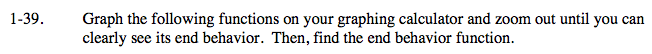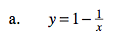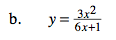### Home > CALC > Chapter 1 > Lesson 1.2.2 > Problem1-39

1-39.Review problem 1-33.

There is a relationship between the end behavior of a function and its horizontal asymptote.There is also a relationship between end behavior and slant or parabolic asymptotes. Before you use long division to compute the end behavior, consider the shape of the graph of this function. Does it have a vertical asymptote, a slant asymptote, or some other type of asymptote? How do the powers of the numerator and denominator help you answer this question?

You should have determined that there will be a slant asymptote, because the numerator is one-degree larger that the denominator. In other words, long division will result in a linear equation of the form:

$y=mx+b+\frac{\text{remainder}}{6x+1}$

The y = mx + b part is the slant asymptote AND the end behavior.

Use the eTool below to view the graphs.
Click the link at right for the full version of the eTool: Calc 1-39 HW eTool